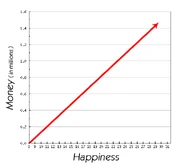## FANDOM

225 PagesDirectly proportional relationship

## About

In mathematics, directly proportional constants are two quantities that are a constant and in the sense of the word moving in the same direction of each other. For example, as one positive number increases, then the other number increases at the same positive rate too. The analogy works for negative numbers too as long as the numbers are moving negatively and in the same negative direction.

In engineering, we use the three fundamental electrical quantities: current, voltage, and resistance to calculate ohms law. We have used Ohm's law to state that the electrical current (I) flowing in an circuit is directly proportional to the voltage (V).

Why? Because if the voltage is increased, we know that the current will increase provided the resistance of the circuit does not change.